How Cheenta works to ensure student success?
Explore the Back-Story

# Test of Mathematics Solution Objective 398 - Complex Number and Binomial TheoremThis is a Test of Mathematics Solution (from ISI Entrance). The book, Test of Mathematics at 10+2 Level is Published by East West Press. This problem book is indispensable for the preparation of I.S.I. B.Stat and B.Math Entrance.

## Problem

If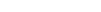are real numbers such that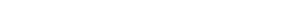for all complex numbers z, then the value of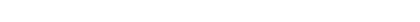equals

(A)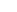; (B)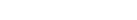; (C)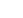; (D)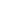;

## Sequential Hints

(How to use this discussion: Do not read the entire solution at one go. First, read more on the Key Idea, then give the problem a try. Next, look into Step 1 and give it another try and so on.)

### Key Idea

This is the generic use case of Complex Number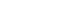and binomial theorem.

### Step 1

Note that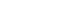. Also, geometrically speaking, i = (0,1). Hence adding (1,0) to i (=(0,1)) gives us the point (1, 1). Polar coordinate of this point is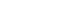. Here is a picture:Try the problem with this hint before looking into step 2. Remember, no one learnt mathematics by looking at solutions.

At Cheenta we are busy with Complex Number and Geometry module. Additionally I.S.I. Entrance Mock Test 1 is also active now.

Replace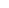by. We have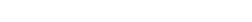on the left hand side.

Now, replacebyon the right hand side.

[/tab]

[tab]

Replacing z byon the right hand side we have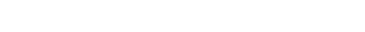. This implies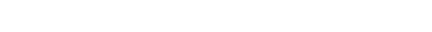Think now, what the following expression represents:It represents the square of the length of point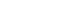. That is simply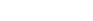Hence the answer is option A.

## More Resources

Look into the following notes on Complex Number and Geometry module.This is a Test of Mathematics Solution (from ISI Entrance). The book, Test of Mathematics at 10+2 Level is Published by East West Press. This problem book is indispensable for the preparation of I.S.I. B.Stat and B.Math Entrance.

## Problem

Ifare real numbers such thatfor all complex numbers z, then the value ofequals

(A); (B); (C); (D);

## Sequential Hints

(How to use this discussion: Do not read the entire solution at one go. First, read more on the Key Idea, then give the problem a try. Next, look into Step 1 and give it another try and so on.)

### Key Idea

This is the generic use case of Complex Numberand binomial theorem.

### Step 1

Note that. Also, geometrically speaking, i = (0,1). Hence adding (1,0) to i (=(0,1)) gives us the point (1, 1). Polar coordinate of this point is. Here is a picture:Try the problem with this hint before looking into step 2. Remember, no one learnt mathematics by looking at solutions.

At Cheenta we are busy with Complex Number and Geometry module. Additionally I.S.I. Entrance Mock Test 1 is also active now.

Replaceby. We haveon the left hand side.

Now, replacebyon the right hand side.

[/tab]

[tab]

Replacing z byon the right hand side we have. This impliesThink now, what the following expression represents:It represents the square of the length of point. That is simplyHence the answer is option A.

## More Resources

Look into the following notes on Complex Number and Geometry module.

### Knowledge Partner